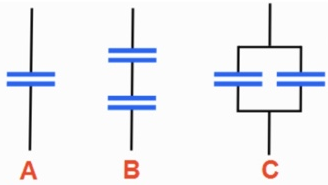# Problem: The three configurations shown below are constructed using identical capacitors1) Which of these configurations has the lowest overall capacitance? a) A b) B c) Cd) All three configurations have the same capacitance 2) Briefly explain your reasoning

###### FREE Expert Solution

Equivalent capacitance in series:

$\overline{)\frac{\mathbf{1}}{{\mathbf{C}}_{\mathbf{e}\mathbf{q}}}{\mathbf{=}}\frac{\mathbf{1}}{{\mathbf{C}}_{\mathbf{1}}}{\mathbf{+}}\frac{\mathbf{1}}{{\mathbf{C}}_{\mathbf{2}}}}$

Equivalent capacitance in parallel:

$\overline{){{\mathbf{C}}}_{\mathbf{e}\mathbf{q}}{\mathbf{=}}{{\mathbf{C}}}_{{\mathbf{1}}}{\mathbf{+}}{{\mathbf{C}}}_{{\mathbf{2}}}}$

(1)

From the equivalent capacitance equations, a series circuit decreases the overall capacitance.

96% (243 ratings)###### Problem Details

The three configurations shown below are constructed using identical capacitors1) Which of these configurations has the lowest overall capacitance?

a) A

b) B

c) C

d) All three configurations have the same capacitance